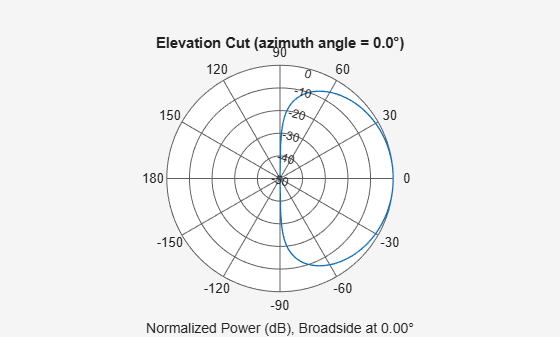## Signal Collection

### Support for Modeling Signal Collection

To model the collection of a signal with a sensor element or sensor array, you can use the `phased.Collector` or `phased.WidebandCollector`. Both collector objects assume that incident signals have propagated to the location of the array elements, but have not been received by the array. In other words, the collector objects do not model the actual reception by the array. See Receiver Preamp for signal effects related to the gain and internal noise of the array’s receiver.

In many array processing applications, the ratio of the signal’s bandwidth to the carrier frequency is small. Expressed as a percentage, this ratio does not exceed a few percent. Examples include radar applications where a pulse waveform is modulated by a carrier frequency in the microwave range. These are narrowband signals. For narrowband signals, you can express the steering vector as a function of a single frequency, the carrier frequency. For narrowband signals, the `phased.Collector` object is appropriate.

In other applications, the narrowband assumption is not justified. In many acoustic and sonar applications, the wave impinging on the array is a pressure wave that is unmodulated. It is not possible to express the steering vector as a function of a single frequency. In these cases, the subband approach implemented in `phased.WidebandCollector` is appropriate. The wideband collector decomposes the input into subbands and computes the steering vector for each subband.

When you use the narrowband collector, `phased.Collector`, you must specify these aspects of the collector:

• Operating frequency of the array

• Propagation speed of the wave

• Sensor (single element) or sensor array

• Type of incoming wave. Choices are `'Plane'` and `'Unspecified'`. If you select `'Plane'`, the input signals are multiple plane waves impinging on the entire array. Each plane wave is received by all collecting elements. If you select `'Unspecified'`, the input signal are individual waves impinging on individual sensors.

• Whether to apply weights to signals collected by different elements in the array. If you want to apply weights, you specify them when you execute the System object™.

When you use the wideband collector, `phased.WidebandCollector`, you must specify these aspects of the collector:

• Carrier frequency

• Whether the signal is demodulated to the baseband

• Operating frequency of the array

• Propagation speed of the wave

• Sampling rate

• Sensor (single element) or sensor array

• Type of incoming wave. Choices are `'Plane'` and `'Unspecified'`. If you select `'Plane'`, the input signals are multiple plane waves impinging on the entire array. Each plane wave is received by all collecting elements. If you select `'Unspecified'`, the input signal are individual waves impinging on individual sensors.

• Whether to apply weights to signals collected by different elements in the array. If you want to apply weights, you specify them when you execute the System object.

### Narrowband Collector for Uniform Linear Array

This example shows how to construct a narrowband collector that models a plane wave impinging on a two-element uniform linear array. The array has an element spacing of 0.5 m (default for a ULA). The operating frequency of the array is 300 MHz.

Create the array and collector System objects.

```array = phased.ULA('NumElements',2,'ElementSpacing',0.5); collector = phased.Collector('Sensor',array,... 'PropagationSpeed',physconst('LightSpeed'),... 'OperatingFrequency',3e8,'Wavefront','Plane');```

Create the signal and simulate reception from an angle of (45;0).

```x =[1 -1 1 -1]'; y = collector(x,[45;0])```
```y = 4×2 complex 0.4433 - 0.8964i 0.4433 + 0.8964i -0.4433 + 0.8964i -0.4433 - 0.8964i 0.4433 - 0.8964i 0.4433 + 0.8964i -0.4433 + 0.8964i -0.4433 - 0.8964i ```

In the preceding case, the collector object multiplies the input signal, `x`, by the corresponding element of the steering vector for the two-element ULA. The following code produces the response in an equivalent manner. First, create the ULA and then create the steering vector. Compare with the previous result.

```array = phased.ULA('NumElements',2,'ElementSpacing',0.5); steeringvec = phased.SteeringVector('SensorArray',array); sv = steeringvec(3e8,[45;0]); x =[1 -1 1 -1]'; y1 = x*sv.'```
```y1 = 4×2 complex 0.4433 - 0.8964i 0.4433 + 0.8964i -0.4433 + 0.8964i -0.4433 - 0.8964i 0.4433 - 0.8964i 0.4433 + 0.8964i -0.4433 + 0.8964i -0.4433 - 0.8964i ```

### Narrowband Collector for a Single Antenna Element

The `Sensor` property of a `phased.Collector` System object™ can specify a single antenna element. In this example, create a custom antenna element using the `phased.CustomAntennaElement` System object. The antenna element has a cosine response over elevation angles from (-90°,90°). Plot the polar pattern response of the antenna at 1 GHz on an elevation cut at 0° azimuth. Display the antenna voltage response at 0° azimuth and 45° elevation.

Note: This example runs only in R2016b or later. If you are using an earlier release, replace each call to the function with the equivalent `step` syntax. For example, replace `myObject(x)` with `step(myObject,x)`.

```fc = 1e9; antenna = phased.CustomAntennaElement; antenna.AzimuthAngles = -180:180; antenna.ElevationAngles = -90:90; antenna.MagnitudePattern = mag2db(... repmat(cosd(antenna.ElevationAngles)',1,numel(antenna.AzimuthAngles))); resp = antenna(fc,[0;45])```
```resp = 0.7071 ```
`pattern(antenna,fc,0,[-90:90],'Type','powerdb')`The antenna voltage response at 0° azimuth and 45° elevation is cos(45°) as expected.

Assume a narrowband sinusoidal input incident on the antenna element from 0° azimuth and 45° elevation. Determine the signal collected at the element.

```collector = phased.Collector('Sensor',antenna,'OperatingFrequency',fc); x =[1 -1 1 -1]'; y = collector(x,[0;45])```
```y = 4×1 0.7071 -0.7071 0.7071 -0.7071 ```

### Wideband Signal Collection

This example shows how to simulate the reception of a wideband acoustic signal by a single omnidirectional microphone element.

Note: This example runs only in R2016b or later. If you are using an earlier release, replace each call to the function with the equivalent `step` syntax. For example, replace `myObject(x)` with `step(myObject,x)`.

```x = randn(10,1); c = 340.0; microphone = phased.OmnidirectionalMicrophoneElement(... 'FrequencyRange',[20 20e3],'BackBaffled',true); collector = phased.WidebandCollector('Sensor',microphone,... 'PropagationSpeed',c,'SampleRate',50e3,... 'ModulatedInput',false); y = collector(x,[30;10]);```